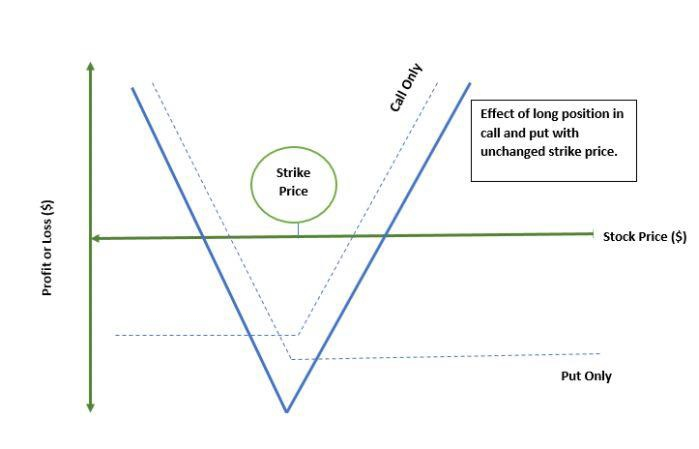# What is a Long Straddle in Investment?

FinanceBanking & FinanceFinance Management

#### TGIF: Transform Grow Innovate the Finance Function

24 Lectures 3 hours

#### Finance for Non Finance Executives

254 Lectures 18 hours

#### Business Finance 101

12 Lectures 1 hours

Long straddle involves call and put strategy for one particular bond, security or an asset and is utilized by traders as it has potential for maximum benefit and negligible risks. Under this method both long call and long put have the same stock price and expiration date.## Important Points Briefly

• An option strategy known as a long straddle is acquiring both a long call and a long put on the same underlying asset with the same expiry date and strike price at the same time.

• The purpose of a long straddle is to benefit from a very big move in either direction by the underlying asset, which is frequently initiated by a newsworthy event.

• The risk associated with a long straddle strategy is that the market may not respond strongly enough to the event or the news that is generated as a result of the event or the news.

• Alternative applications for the long straddle strategy include capturing the projected rise in implied volatility, which would grow if the demand for these options grows in the short term.

## Long Straddle - Example of StocksLet us take the example of stock that is priced at $60 per share. The call and put option for this share of$60 is priced at $3. When the investor enters the long straddle they will have to calculate all options and probability that might happen with them during the day. If it is assumed that there will be a swing in price of the share of$6 increasing or lowering the price value, then the trader will be left at $66 incase of an upward curve or a$54 incase or reduction.

When the price stays constant at $60, the biggest, Max Loss for$6 per share price could be at $600. This is calculated using the formula − (Call premium + Put premium) × 100 However, when the price of share fluctuates between$66 & $54 there will be marginally smaller loss when compared to other scenarios. If the stock closed the day at$80, the profit margin will be calculated as below −

Profit= $80 -$65 - $6 =$9

## How to use Long Straddle?

There is negligible risk in long straddle usage and potential for maximum gain. It is possible to gain an indefinite amount of money by investing in an underlying asset that is increasing in value. For a zero-price underlying asset, the profit would equal the strike price minus any premiums paid on the options. No matter which option you choose, the maximum risk is equal to the cost of entering the position, which equals the sum of the call option's price plus the price of the put option's price.

When the price of the underlying asset rises, the profit is provided by the following equation −

Profit (up) = Price of underlying asset – strike price of call option – net premium paid

When there is a reduction in the price of the underlying asset, we utilize the below formula −

Profit (down) = Strike price put option - the price of underlying asset - net premium paid